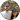HomeThis script is used for compiling a total of all purchases from My eBay: Purchase history.

It is posted here so we can keep track of it, not for the purpose of others to use it.

Disclaimer: I take no responsibility if you use this script. Ebay can change their website and break the script at any time, or I could be adding numbers other than you expect.

`.css-1baulvz{display:inline-block;}function ebayTotals() {  if (typeof window.itemTotals === "undefined") {    window.itemTotals = []  }  var currentPageTotal = 0  document.querySelectorAll(".order-r").forEach(function(orderRow) {    // This will find the first cost label, which is either the "order total" or    // the item total if it is the only number.    var costLabel = orderRow.querySelector(".cost-label")    currentPageTotal += parseInt(costLabel.innerText.replace(/[^\d]/g, ""))  })  window.itemTotals.push(currentPageTotal)  var message = ""  message += "You have added the totals for "  message += window.itemTotals.length.toString() + " pages so far:"  message += "\n"  message += "\n"  window.itemTotals.forEach(function(pageTotal, index) {    message += "page " + (index + 1) + ": "    message += pageTotal.toString().replace(/(\d+?)(\d{2})\$/, "\$1.\$2")    message += "\n"  })  message += "-----------------------"  message += "\n"  message += "Your current total is: "  message += window.itemTotals    .reduce((a, e) => a + e)    .toString()    .replace(/(\d+?)(\d{2})\$/, "\$1.\$2")  message += "\n"  message += "\n"  message += "Go to the next page and run this script again to continue adding."  alert(message)}`

Here is is as a bookmarklet:

`javascript:(function ebayTotals(){if(typeof window.itemTotals==="undefined"){window.itemTotals=[]}var currentPageTotal=0;document.querySelectorAll(".order-r").forEach(function(orderRow){var costLabel=orderRow.querySelector(".cost-label");currentPageTotal+=parseInt(costLabel.innerText.replace(/[^\d]/g,""))});window.itemTotals.push(currentPageTotal);var message="";message+="You have added the totals for ";message+=window.itemTotals.length.toString()+" pages so far:";message+="\n";message+="\n";window.itemTotals.forEach(function(pageTotal,index){message+="page "+(index+1)+": ";message+=pageTotal.toString().replace(/(\d+?)(\d{2})\$/,"\$1.\$2");message+="\n"});message+="-----------------------";message+="\n";message+="Your current total is: ";message+=window.itemTotals.reduce((a,e)=>a+e).toString().replace(/(\d+?)(\d{2})\$/,"\$1.\$2");message+="\n";message+="\n";message+="Go to the next page and run this script again to continue adding.";alert(message)})();`